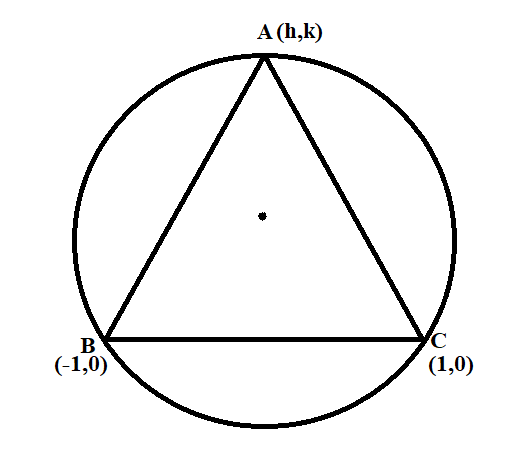QuestionAnswers

# Three distinct points A,B and C are given in the 2-dimensional coordinate plane such that the ratio of the distance of any point from the point $\left( {1,0} \right)$ to the distance from the point $\left( { - 1,0} \right)$ is equal to $\dfrac{1}{3}$. Then the circumcentre of the triangle ABC is at point:A.$\left( {\dfrac{5}{4},0} \right)$B.$\left( {\dfrac{5}{2},0} \right)$C.$\left( {\dfrac{5}{3},0} \right)$D.$\left( {0,0} \right)$

Hint: We will first let point be $\left( {h,k} \right)$ whose distance from the point $\left( {1,0} \right)$ to the distance from the point $\left( { - 1,0} \right)$ is equal to $\dfrac{1}{3}$. Find the distance of both the given points from $\left( {h,k} \right)$ and use the given ratio to form the equation of circumcircle which passes through the vertices of the triangle ABC. Compare the equation with the standard equation of the circle to find the circumcentre.

Let the coordinates of the point be $\left( {h,k} \right)$ whose distance from the point $\left( {1,0} \right)$ to the distance from the point $\left( { - 1,0} \right)$ is equal to $\dfrac{1}{3}$.We will first find the distance from $\left( {h,k} \right)$ to $\left( {1,0} \right)$
If $\left( {{x_1},{y_1}} \right)$ and $\left( {{x_2},{y_2}} \right)$ are two points, then the distance between the points is given by $\sqrt {{{\left( {{x_2} - {x_1}} \right)}^2} + {{\left( {{y_2} - {y_1}} \right)}^2}}$
Then, the distance from $\left( {h,k} \right)$ to $\left( {1,0} \right)$ will be $\sqrt {{{\left( {h - 1} \right)}^2} + {k^2}}$
Similarly, the distance from $\left( {h,k} \right)$ to $\left( { - 1,0} \right)$ will be $\sqrt {{{\left( {h + 1} \right)}^2} + {k^2}}$
We are given that the ratio of both the distances is $\dfrac{1}{3}$
Hence, $\dfrac{{\sqrt {{{\left( {h - 1} \right)}^2} + {k^2}} }}{{\sqrt {{{\left( {h + 1} \right)}^2} + {k^2}} }} = \dfrac{1}{3}$
On squaring both sides, we will get,
$\dfrac{{{{\left( {h - 1} \right)}^2} + {k^2}}}{{{{\left( {h - 1} \right)}^2} + {k^2}}} = \dfrac{1}{9}$
Cross-multiply and simplify
$9\left( {{{\left( {h - 1} \right)}^2} + {k^2}} \right) = {\left( {h - 1} \right)^2} + {k^2} \\ \Rightarrow 9\left( {{h^2} + 1 - 2h + {k^2}} \right) = {h^2} + 1 + 2h + {k^2} \\$
On solving the brackets, we get
$9\left( {{h^2} + 1 - 2h + {k^2}} \right) = {h^2} + 1 + 2h + {k^2} \\ \Rightarrow 9{h^2} + 9 - 18h + 9{k^2} = {h^2} + 1 + 2h + {k^2} \\ \Rightarrow 8{h^2} + 8{k^2} - 20h + 8 = 0 \\ \Rightarrow {h^2} + {k^2} - \dfrac{5}{2}h + 1 = 0 \\$
We have to find the circumcentre of the triangle ABC.
Circumference is the centre of the circle which passes through each point on the circle.
The equation ${h^2} + {k^2} - \dfrac{5}{2}h + 1 = 0$ represents the equation of the circle.
Compare it with the standard equation of the circle to find the circumcentre.
The standard equation of the circle is ${x^2} + {y^2} + 2gx + 2fy + c = 0$, whose centre is $\left( { - g, - f} \right)$
Here, $2g = - \dfrac{5}{2}$ and $2f = 0$
Hence, $g = - \dfrac{5}{4}$ and $f = 0$
Therefore, the coordinates of circumcentre is $\left( {\dfrac{5}{4},0} \right)$
Hence, option A is correct.

Note: Since, we have to find the circumcentre of the triangle with vertices A,B and C, the coordinates of any vertex A,B or C will satisfy the equation of the circumcircle. Also, the general equation of circle is ${x^2} + {y^2} + 2gx + 2fy + c = 0$, where $\left( { - g, - f} \right)$ is the centre of the circle and $\sqrt {{g^2} + {f^2} - c}$ is the radius of the circle.